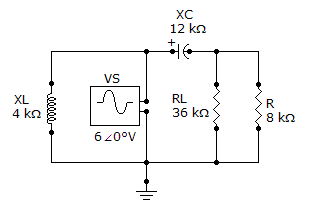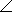# Electrical Engineering - Circuit Theorems in AC Analysis - Discussion

### Discussion :: Circuit Theorems in AC Analysis - General Questions (Q.No.3)

3.

Referring to the given circuit, find ZTH if R is 15 kand RL is 38 k.[A]. 89.82–51.3° k[B]. 19.2–38.3° k[C]. 9.38–51.3° k[D]. 180–38.3° kExplanation:

No answer description available for this question.

 Shashwat said: (Sep 1, 2011) Its not provided in the question that the Zth has to be found across which branch.

 Nikhil Jain said: (Jun 29, 2014) The parallel combination of j12 and 15 will give the answer.

 Vasiq said: (Jan 18, 2015) 15? You mean 8k?

 Richa Dhingra said: (Apr 15, 2015) Zth is found across load so RL will open circuit and voltage source short circuit so XL will also SC. So XC and are will become parallel to each other and gave answer.

 Ali Mohamed said: (Jul 8, 2015) @Vasiq he said to replace 8K with 15K.

 Chinni Krishna said: (Mar 5, 2016) Can anyone tell which is the correct answer.

 Abdi said: (Apr 21, 2016) First short the Vs. Since Vs is in parallel with Xl, Xl goes to zero. Second remove the Rl by opening it.that is the point where Zth is found. Now R (8K) is in parallel with Xc. Zth = Xc//R = j12*15 / (j12 + 15) = 9.37

 Ahmad Ado said: (Jul 28, 2016) How to work out the solution?

 Rajeeb Giri said: (Jun 28, 2017) Can tell which is the correct answer?

 Sashank said: (Mar 11, 2019) The answer is 9.3θ +51.3°.